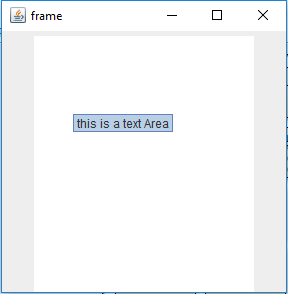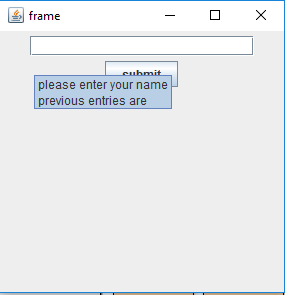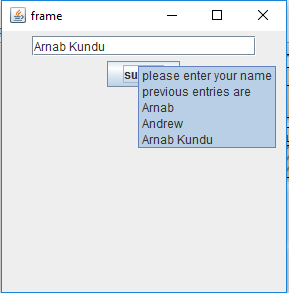# Java Swing | ToolTip

We can add tooltip text to almost all the components of Java Swing by using the following method setToolTipText(String s). This method sets the tooltip of the component to the specified string s . When the cursor enters the boundary of that component a popup appears and text is displayed .

Methods used:

1. getToolTipText() : returns the tooltip text for that component .
2. setToolTipText(String s) : sets the tooltip text for the component .
3. getToolTipText(MouseEvent e): returns the same value returned by getToolTipText(). Multi-part components such as JTabbedPane, JTable, and JTree override this method to return a string associated with the mouse event location.
4. getToolTipLocation(MouseEvent e) : Returns the location (in the receiving component’s coordinate system) where the upper left corner of the component’s tool tip appears.

The following programs will illustrate the use of tooltiptext
1. Program to create a textarea and single line tool tip text to it

 `// java Program to create a textarea and ` `// single line tool tip text to it ` `import` `javax.swing.event.*; ` `import` `java.awt.*; ` `import` `javax.swing.*; ` `class` `solve ``extends` `JFrame { ` ` `  `    ``// frame ` `    ``static` `JFrame f; ` ` `  `    ``// text areas ` `    ``static` `JTextArea t1; ` ` `  `    ``// main class ` `    ``public` `static` `void` `main(String[] args) ` `    ``{ ` `        ``// create a new frame ` `        ``f = ``new` `JFrame(``"frame"``); ` ` `  `        ``// create a object ` `        ``solve s = ``new` `solve(); ` ` `  `        ``// create a panel ` `        ``JPanel p = ``new` `JPanel(); ` ` `  `        ``// create a text area ` `        ``t1 = ``new` `JTextArea(``20``, ``20``); ` ` `  `        ``// set tooltip text ` `        ``t1.setToolTipText(``"this is a text Area"``); ` ` `  `        ``// add text area ` `        ``p.add(t1); ` ` `  `        ``// add panel ` `        ``f.add(p); ` ` `  `        ``// set the size of frame ` `        ``f.setSize(``300``, ``300``); ` ` `  `        ``f.show(); ` `    ``} ` `} `2. Program to create a text area and add multiple line tooltip text to it.

 `// java Program to create a text area and add ` `// multiple line tooltip text to it. ` `import` `javax.swing.event.*; ` `import` `java.awt.*; ` `import` `javax.swing.*; ` `class` `solve ``extends` `JFrame { ` ` `  `    ``// frame ` `    ``static` `JFrame f; ` ` `  `    ``// text areas ` `    ``static` `JTextArea t1; ` ` `  `    ``// main class ` `    ``public` `static` `void` `main(String[] args) ` `    ``{ ` `        ``// create a new frame ` `        ``f = ``new` `JFrame(``"frame"``); ` ` `  `        ``// create a object ` `        ``solve s = ``new` `solve(); ` ` `  `        ``// create a panel ` `        ``JPanel p = ``new` `JPanel(); ` ` `  `        ``// create a text area ` `        ``t1 = ``new` `JTextArea(``20``, ``20``); ` ` `  `        ``// create a multi line string using html using break tags ` `        ``String s1 = ``" this is a text area
it has 20 rows
it has 20 columns "``; ` ` `  `        ``// set tooltip text ` `        ``t1.setToolTipText(s1); ` ` `  `        ``// add text area ` `        ``p.add(t1); ` ` `  `        ``// add panel ` `        ``f.add(p); ` ` `  `        ``// set the size of frame ` `        ``f.setSize(``300``, ``300``); ` ` `  `        ``f.show(); ` `    ``} ` `} `

Output:3. program to submit name using JTextField and the tooltip text shows the previous entries.(using getToolTipText function)

 `// java Program to submit name using JTextField and the tooltip ` `// text shows the previous entries.(using ` `// getToolTipText function) ` `import` `java.awt.event.*; ` `import` `java.awt.*; ` `import` `javax.swing.*; ` `class` `solve ``extends` `JFrame ``implements` `ActionListener { ` ` `  `    ``// frame ` `    ``static` `JFrame f; ` ` `  `    ``// text areas ` `    ``static` `JTextField t1; ` ` `  `    ``// buttons ` `    ``static` `JButton b; ` ` `  `    ``// main class ` `    ``public` `static` `void` `main(String[] args) ` `    ``{ ` `        ``// create a new frame ` `        ``f = ``new` `JFrame(``"frame"``); ` ` `  `        ``// create a object ` `        ``solve s = ``new` `solve(); ` ` `  `        ``// create a panel ` `        ``JPanel p = ``new` `JPanel(); ` ` `  `        ``// create a text area ` `        ``t1 = ``new` `JTextField(``20``); ` ` `  `        ``// create a button ` `        ``b = ``new` `JButton(``"submit"``); ` ` `  `        ``// add actionlistener ` `        ``b.addActionListener(s); ` ` `  `        ``// create a multi line string using html using break tags ` `        ``String s1 = ``" please enter your name
previous entries are
"``; ` ` `  `        ``// set tooltip text ` `        ``t1.setToolTipText(s1); ` ` `  `        ``// add text areaa nd button ` `        ``p.add(t1); ` `        ``p.add(b); ` ` `  `        ``// add panel ` `        ``f.add(p); ` ` `  `        ``// set the size of frame ` `        ``f.setSize(``300``, ``300``); ` ` `  `        ``f.show(); ` `    ``} ` ` `  `    ``// if a button is performed ` `    ``public` `void` `actionPerformed(ActionEvent e) ` `    ``{ ` `        ``// if submit button is pressed add the name to the list of entries ` `        ``// excude the closing html tag bu taking its substring ` `        ``// add the mane to tle list of entries ` `        ``// and add the html tag to the end of it ` ` `  `        ``// get the tooltip text ` `        ``String s = t1.getToolTipText(); ` ` `  `        ``t1.setToolTipText(s.substring(``0``, s.length() - ``8``) + t1.getText() + ``"

Output :Note : the following programs might not run in an online compiler please use an online IDE.

Attention reader! Don’t stop learning now. Get hold of all the important Java and Collections concepts with the Fundamentals of Java and Java Collections Course at a student-friendly price and become industry ready.

My Personal Notes arrow_drop_upCheck out this Author's contributed articles.

If you like GeeksforGeeks and would like to contribute, you can also write an article using contribute.geeksforgeeks.org or mail your article to contribute@geeksforgeeks.org. See your article appearing on the GeeksforGeeks main page and help other Geeks.

Please Improve this article if you find anything incorrect by clicking on the "Improve Article" button below.

Improved By : ManasChhabra2

Article Tags :
Practice Tags :

Be the First to upvote.

Please write to us at contribute@geeksforgeeks.org to report any issue with the above content.